# Linear transformation

(diff) ← Older revision | Latest revision (diff) | Newer revision → (diff)

A mapping of a vector space into itself under which the image of the sum of two vectors is the sum of their images and the image of the product of a vector by a number is the product of the image of the vector by this number. Ifis a vector space,is a linear transformation defined in it,are any vectors of the space, andis any number (an element of a field), then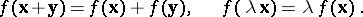If a vector spacehas finite dimension,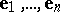is a basis of it,are the coordinates of an arbitrary vector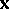in this basis, and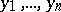are the coordinates of its image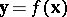, then the coordinates of the vectorare expressed in terms of the coordinates of the vectorby linear homogeneous functions: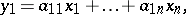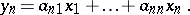The matrix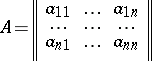is called the matrix of the linear transformationin the basis. Its columns consist of the coordinates of the images of the basis vectors. Ifis the transition matrix from the basisto a basis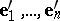: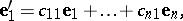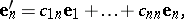then in the basisthe matrixof the linear transformationis.

The sum of two linear transformationsandis the transformationsuch that for any vector,The product of a linear transformationby a numberis the transformationfor which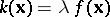for every vector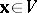.

The product of a linear transformationby a linear transformationis the transformation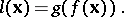The sum of two linear transformations, the product of a linear transformation by a number, and the product of two linear transformations (in any order) are themselves linear transformations. The linear transformations form an algebra. In the case a finite-dimensional space of dimension, the algebra of its linear transformations is isomorphic to the algebra of square matrices of orderwith as entries the elements of the field over which the vector space is constructed.

A linear transformationunder which a vector space is mapped onto itself is said to be invertible if there is a transformation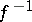such that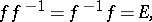whereis the identity transformation. The transformationis a linear transformation and is called the inverse transformation of. A linear transformation defined on a finite-dimensional vector space is invertible if and only if the determinant of its matrix in some (and therefore in any) basis is non-zero. Ifis the matrix of an invertible linear transformation, then the matrix of the inverseis. The invertible linear transformations form a group with respect to multiplication. In the case of a vector space of finite dimension, this group is isomorphic to the group of non-singular square matrices of order.

A subspaceof a vector spaceis called an invariant subspace with respect to a linear transformationiffor every vector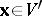. A non-zero vectoris called an eigen vector of a linear transformation, corresponding to the eigen value, if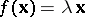. In the case of a finite-dimensional space over the field of complex numbers (or, more generally, an algebraically closed field) every linear transformation has an eigen vector (a one-dimensional invariant subspace). In the case of a finite-dimensional space over the field of real numbers every linear transformation has a one-dimensional or two-dimensional invariant subspace.

A linear transformation, defined on a finite-dimensional vector space, is called a diagonalizable linear transformation if there is a basis inin which the matrix of this transformation has diagonal form (cf. Diagonal matrix). In other words, a linear transformation is diagonalizable if the space has a basis consisting of eigen vectors of this linear transformation. However, not every linear transformation has a basis of eigen vectors even in a space over the field of complex numbers. E.g. the linear transformation of a two-dimensional space given by the matrix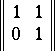has a unique one-dimensional invariant subspace with basis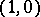.

In a finite-dimensional vector space over the field of complex numbers (or any algebraically closed field) there is for every linear transformation a basis in which the matrix of this transformation has block form (cf. Block-diagonal operator), with Jordan blocks on the main diagonal and zeros elsewhere. A Jordan block of the first order consists of one number; a Jordan block of orderis a square matrix of orderof the form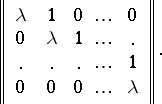The numbersare the eigen values of the matrix of the linear transformation. To one and the sameseveral blocks of the same order may correspond, as well as blocks of different orders. The matrix consisting of Jordan blocks is called the Jordan normal form (or Jordan canonical form) of the matrix.

A linear transformation, defined on a Euclidean (unitary) space (cf. Unitary space), is said to be self-adjoint (respectively, Hermitian) if for any two vectors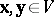one has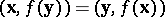(respectively,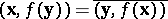).

A linear transformation, defined on a finite-dimensional Euclidean (unitary) space, is self-adjoint (Hermitian) if and only if its matrixin some (and therefore any) orthonormal basis is symmetric (respectively, Hermitian, cf. Hermitian matrix; Symmetric matrix). A self-adjoint (Hermitian) linear transformation, defined on a finite-dimensional Euclidean (respectively, unitary) space, has an orthonormal basis in which its matrix has diagonal form. The main diagonal consists of the (always real) eigen values of the matrix.

A linear transformation, defined on a Euclidean (unitary) space, is said to be isometric or orthogonal (respectively, unitary) if for every vector,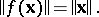A linear transformation, defined on a finite-dimensional Euclidean (unitary) space, is isometric (respectively, unitary) if and only if its matrixin some (and then in any) orthonormal basis is orthogonal (respectively, unitary, cf. Orthogonal matrix; Unitary matrix). For every isometric linear transformation, defined on a finite-dimensional Euclidean space, there is an orthonormal basis in which the matrix of the transformation consists of blocks of the first and second orders on its main diagonal. The blocks of the first order are the real eigen values of the matrixof the transformation, equal toand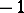, and the blocks of the second order have the form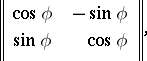whereandare the real and imaginary parts of the complex eigen value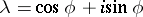of, and the other entries ofare zero. For every unitary transformation, defined on a unitary space, there is an orthonormal basis in which the matrix of this transformation is diagonal and on the main diagonal there are numbers of absolute value 1.

Every linear transformation, defined on a finite-dimensional Euclidean (unitary) space, is the product of a self-adjoint (cf. Self-adjoint linear transformation) and an isometric linear transformation (respectively, of a Hermitian and a unitary linear transformation).

How to Cite This Entry:
Linear transformation. Encyclopedia of Mathematics. URL: http://encyclopediaofmath.org/index.php?title=Linear_transformation&oldid=15932
This article was adapted from an original article by A.S. Parkhomenko (originator), which appeared in Encyclopedia of Mathematics - ISBN 1402006098. See original article